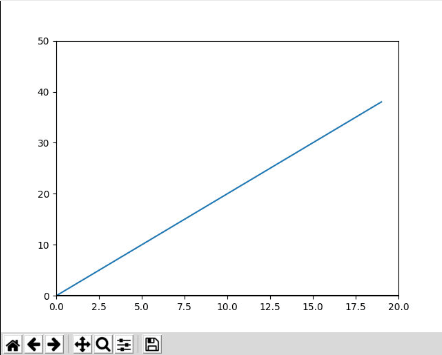# Programming with Python – Class 11

## Graphics

We can extend the Python functionalities with a series of libraries or packages, in this and subsequent classes we will see some interesting libraries and how to use them.

Libraries usually need to be loaded first and then import a reference to them into the program.

In the Repl.it environment we can add the library in the “Packages” option, search for the library that we want to install and press the + button

### Matplotlib

It is a library to visualize 2D and 3D graphics

To better follow how it’s done, I’ll give an example

We want to draw the graph of the function f (x) = 2x

The code to make the graph could be something like

```# - * - coding: utf-8 - * -
# Example python 3
# Show the graph of the functions on the screen
# f2 (x) = 2x
# where x> = 0 and x <= 20

#import the graphics processing library
from matplotlib import pyplot

# Definition of functions
def f (x):
return 2 * x

if __name__ == "__main__":
# Values ​​of the X axis taken by the graph.
x = range (0, 20)

# Graph function
pyplot.plot (x, [f (i) for i in x])

# Set the color of the axes.
pyplot.axhline (0, color = "black")
pyplot.axvline (0, color = "black")

# Limit the values ​​of the axes.
pyplot.xlim (0, 20)

pyplot.ylim (0, 50)

# Save graphic as PNG image.
pyplot.savefig ("output.png")

# Show it.
pyplot.show ()

```

To draw a graph we must import the library with the instruction

from matplotlib import pyplot

To draw the graph of a function we will use

x = range (0, 20)

pyplot.plot (x, [f (i) for i in x])

The second parameter of the plot function is the result of applying a function to each of the points defined in the range of x.

This type of constructs may seem strange to programmers more used to imperative languages ​​like C and another type of paradigm called Functional programming is followed in which functions can be defined dynamically.

Normally a graph is generated and saved in a file (savefig) and after displayed on the screen (show)

The result of the previous example would be something like